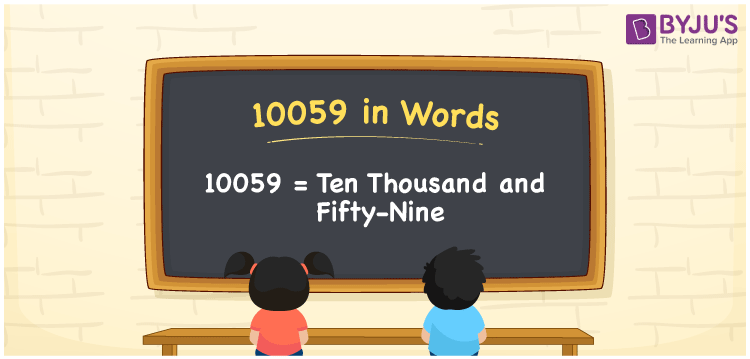# 10059 in Words

10059 in words is Ten Thousand and Fifty-Nine. For instance, Rashmi purchased a room heater worth Rs. 10059, then you can say, “Rashmi purchased a room heater worth Rupees Ten Thousand and Fifty-Nine”. As we know 10059 is a cardinal number as it denotes a certain amount. Using a place value table we can convert the number into words effortlessly. In this article, we will learn how to convert the number 10059 into words in a comprehensive manner.

 10059 in Words Ten Thousand and Fifty-Nine Ten Thousand and Fifty-Nine in numerical form 10059

## 10059 in English Words

English is the most used language in the education field. Hence, we write the numbers in words using the letters of the English alphabet. Therefore, we can write the number 10059 in English words as Ten Thousand and Fifty-Nine.## How to Write 10059 in Words?

10059 is a five-digit number. So, we need a place value chart with 5 columns. The place value chart for the number 10059 is shown below.

 Ten-Thousands Thousands Hundreds Tens Ones 1 0 0 5 9

Therefore, we can write the expanded form as:

1 x Ten Thousand + 0 x Thousand + 0 x Hundred + 5 x Ten + 9 x One

= 1 x 10000 + 0 x 1000 + 0 x 100 + 5 x 10 + 9 x 1

= 10000 + 0 + 0 + 50 + 9

= 10000 + 50 + 9

= 10059

= Ten Thousand and Fifty-Nine

Hence, 10059 in words is written as Ten Thousand and Fifty-Nine

Interesting way of writing 10059 in words

1 = One

10 = Ten

100 = Hundred

1005 = One Thousand Five

10059 = Ten Thousand and Fifty-Nine

Thus, the word form of the number 10059 is Ten Thousand and Fifty-Nine

10059 is a natural number that precedes 10060 and succeeds 10058

• 10059 in words – Ten Thousand and Fifty-Nine
• Is 10059 an odd number? – Yes
• Is 10059 an even number? – No
• Is 10059 a perfect square number? – No
• Is 10059 a perfect cube number? – No
• Is 10059 a prime number? – No
• Is 10059 a composite number? – Yes

## Frequently Asked Questions on 10059 in Words

Q1

### How do you write 10059 in words?

We write 10059 in words as Ten Thousand and Fifty-Nine.
Q2

### Express the value of 9000 + 1059 in word form.

9000 + 1059 = 10059. Therefore, the value of 9000 + 1059 i.e., 10059 in words is Ten Thousand and Fifty-Nine.
Q3

### How do you write Ten Thousand and Fifty-Nine in numbers?

Ten Thousand and Fifty-Nine in numbers is 10059.